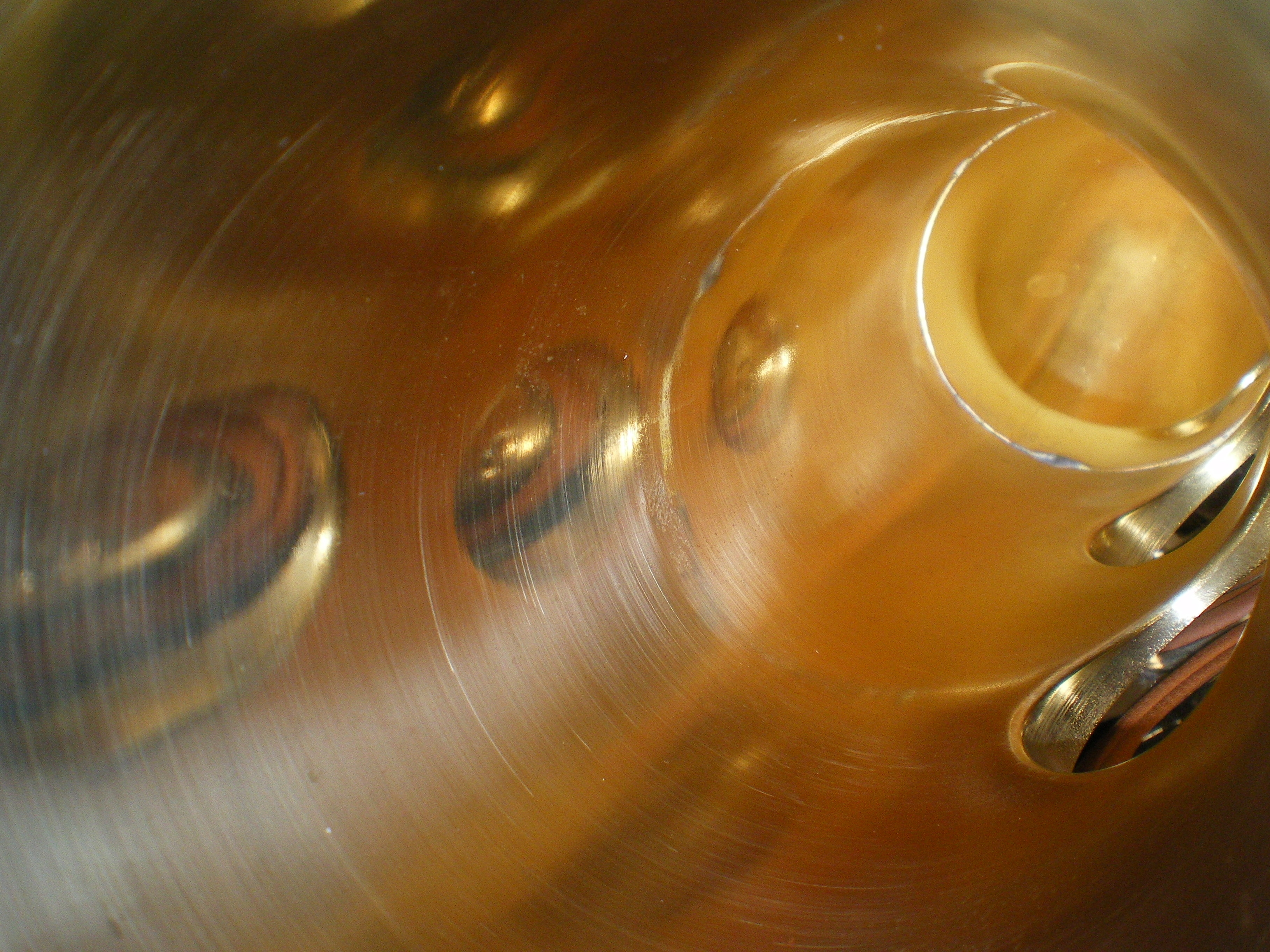##Distributed Systems and Networks

Information and Computation

Stochastic Processes

Geometry

## Stochastic Processes

Classical probability theory for martingales is traditionally based upon a probability space (Ω, F, m) consisting of a sample space Ω, a sigma algebra of subspaces of the sample space and a probability measure m together with a filtration { Ft}consisting of complete sigma - subrings of F. Conditional expectation operators E ( . | Ft ) are also employed mapping functions integrable with respect to F to functions integrable with respect to Ft. A martingale is then a process Xt such that " t' ≥ t, E (Xt'| Ft) = Xt. Reformulating the theory in terms of the Hilbert space L2(Ω, F , m) with associated filtration L∞(Ω, Ft , m) (a von Neumann algebra filtration) and conditional expectation (projection) from L2(Ω, F , m) to L2(Ω, Ft , m) one may develop a quantum analogue of the classical construction based for example on Irving Segals probability gage space (H, A, m) consisting of a Hilbert space H, a von Neumann algebra A, a filtration of von Neumann algebras { A z} z and gage m. We consider non-commutative constructions in Clifford and Quasi-Free settings resulting in orthogonal, centred L2 martingales, obeying isometry properties as stochastic integrals. Representation theorems for such martingales have been established and research is actively being pursued in this and related areas of probability theory.

Publications
W. J. Spring, Martingale Representation in the Clifford and Quasi-Free Sheet, Quantum Communication, Measurement and Computing 8, AIP, (2007)
W. J. Spring AND I. F. Wilde, Quasi-Free Quantum Stochastic Integrals in the Plane, Rep. Math. Phys. 49 (2002), 63-76